Courses

# Competition Level Test: Alternating Current

## 30 Questions MCQ Test Physics Class 12 | Competition Level Test: Alternating Current

Description
This mock test of Competition Level Test: Alternating Current for Class 12 helps you for every Class 12 entrance exam. This contains 30 Multiple Choice Questions for Class 12 Competition Level Test: Alternating Current (mcq) to study with solutions a complete question bank. The solved questions answers in this Competition Level Test: Alternating Current quiz give you a good mix of easy questions and tough questions. Class 12 students definitely take this Competition Level Test: Alternating Current exercise for a better result in the exam. You can find other Competition Level Test: Alternating Current extra questions, long questions & short questions for Class 12 on EduRev as well by searching above.
QUESTION: 1

### In an ac circuit, peak value of voltage is 423 volts. Its effective voltage is

Solution:

Effective voltage is also known as root mean square value of voltage of alternating current in an AC circuit.
we know, Erms=Epeak/ √2
where Erms  denotes the rms voltage and Epeak peak voltage in an ac circuit.
here, peak voltage , Epeak =423 volts
so, effective voltage , Erms  =423/√2  ≈ 300 volts
hence, effective voltage is 300 volts
The correct answer is option C.

QUESTION: 2

### An ac circuit, the current is given by i = 4 sin (100pt + 30º) ampere. The current becomes maximum first time (after t = 0) at t equal to

Solution:

i=4sin(100πt+ π/6)
For I to be maximum
di/dt=0
therefore,4x100π(cos(100πt+π/6)=0
therefore, ωs(100πt+ π/6)=0
for the first time cosθ=0 at π/2
⇒ 100πt+ π/6= π/2
⇒ 100πt= π/3
⇒ πt= π/300
T=1/300

QUESTION: 3

### The instantaneous value of current in an ac circuit is I = 2 sin (100pt + p/3) A. The current at the beginning (t = 0) will be

Solution:

Instantaneous current i=2sin(100πt+π/3)A
At (t=0),  i(0)=2sin(π/3)=2×√3/2​​=√3​A
The current at the beginning will be √3​ A

QUESTION: 4

If instantaneous value of current is I = 10 sin (314 t) A, then the average current for the half cycle will be

Solution:

I=10sin(314t)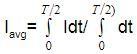dt      [ω=314, T=2π/​ω=2π/​314, T=π/​157]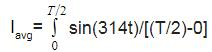Iavg=(2/T)x10 [-cos314/314]T/2
=-20/(Tx314)[cos{314x(π/2x157)}-cos0]
=20/3.14
=6.37A

QUESTION: 5

In a circuit an a.c. current and a d, c. current are supplied together. The expression of the instantaneous current is given as i = 3 + 6 sin wt. Then the rms value of the current is

Solution:

Given,
Instantaneous current, i=3+6sinωt
irms​=√[(1/T​)0T(3+6sinωt)2dt​]
irms​=√(1/T) 0T​(32+62sin2ωt+36sinωt) dt​
irms​=√(1/T​) [0T32dt+∫0T​62{(1/2) ​– (cos2ωt/2​) dt+∫0T​(36sinωt)dt]​
irms​=√9+(36/2) ​+0​=3√3​A
Hence, RMS value of current is 3√3​A

QUESTION: 6

The emf and the current in a circuit are E = 12 sin (100pt) ; I = 4 sin (100pt + p / 3) then

Solution:
QUESTION: 7

If the frequency of alternating potential is 50Hz then the direction of potential, changes in one second by

Solution: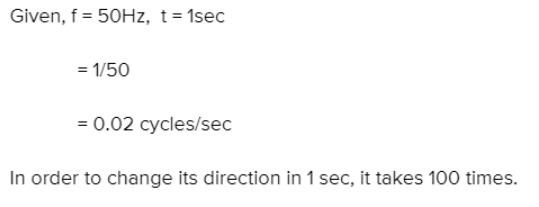QUESTION: 8

The value of alternating e.m.f. is e = 500 sin 100pt , then the frequency of this potential in Hz is

Solution:

l=500sin(100πt)
⟹ω=100π
ω=2πf
⟹2πf=100π
f=50Hz

QUESTION: 9

The domestic power supply is at 220 volt. The amplitude of emf will be

Solution:

We know: Vrms​=​Vo​​/√2

Substituting values (Here RMS Voltage is 220 V)
We get: Maximum V (or amplitude) =311V

QUESTION: 10

The average value or alternating current for half cycle in terms of I0 is

Solution:

For alternating current average value is taken for half cycles only,
Let, I=I0sinωt where ω=2π/T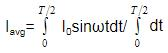=[(I0/ω) cosωt]oT/2 x 2/T
Iavg=2Io/ π

QUESTION: 11

Sinusoidal peak potential is 200 volt with frequency 50Hz. It is represented by the equation

Solution:

Given peak potential is 200v
so, amplitude is 200
and frequency is 50Hz
so angular frequency is 2.πx50 = 314/sec
so the value is
E = 200sin(314t)
Hence option B is the correct answer.

QUESTION: 12

RMS value of ac i = i1 cos wt + i2 sin wt will be

Solution:

I=(I1)cosωt+(I2)sinωt
(I2)mean=I12cos2ωt+I22sin2ωt+I1I2cosωtsinωt
I12×(1/2)×i22×(1/2)+2I1I2×0
Irms=√(i2)mean=√(I12+I22/2)=(1/√2)( I12+I22)1/2

QUESTION: 13

The phase difference between the alternating current and voltage represented by the following equation I = I0 sin wt, E = E0 cos (wt + p / 3), will be

Solution:

E= εocos(ωt+π/3) –1
I=I0sin(ωt)=I0cos{(π/2)-cot)
=I0cos(ωt+(- π/2)) —2
By,1 and 2,
Phase difference between E and I,
E-VI
=(ωt+π/3)- (ωt+(- π/2))
= π/3+ π/2
=5π/6

QUESTION: 14

The inductive reactance of a coil is 1000W. If its self inductance and frequency both are increased two times then inductive reactance will be

Solution:

We know that,
XL= ωL
Or, XL =2πfL   [ ω can be written as, 2πf]
Initially,
XL1=2πf1L1=1000Ω
For the second case,
F  and L both are doubled, so,
XL2 =2π2f12L1 =4x2πf1L1
=4000 Ω

QUESTION: 15

In an L.C.R series circuit R = 1W, XL = 1000W and XC = 1000W. A source of 100 m.volt is connected in the circuit the current in the circuit is

Solution: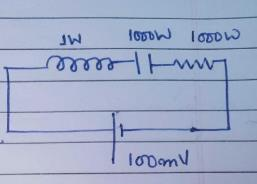R=1W
XL=1000W
XC=1000W
Z= Z= √ (R2+(XL-XC)2)
=√(12+(1000-1000)2)
Z=1W
I=V/Z=100/1
I=1000A

QUESTION: 16

A coil of inductance 0.1 H is connected to an alternating voltage generator of voltage E = 100 sin (100t) volt. The current flowing through the coil will be

Solution:

L =0.14
E=100sin(100t)
⇒XL​=wL​=100×0.1
=10Ω
⇒io​=Eo/XL​=100/10​=10A
⇒i=io​sin(100t− π/2​)
⇒i=−io​sin[(π/2)​−100]
So we get
⇒i=−10cos(100t)A
therefore, option D is correct.

QUESTION: 17

Alternating current lead the applied e.m.f. by p/2 when the circuit consists of

Solution:
QUESTION: 18

A coil has reactance of 100W when frequency is 50Hz. If the frequency becomes 150Hz, then the reactance will be

Solution:

Given,
Reactance of a coil at 50 Hz, XL=100ohm
If frequency becomes 150 Hz, let the reactance be XL
As, XL= ωL
=2πfL
XL=2π(50)L……(1)
XL’=2π(150)L…….(2)
Dividing 2 by 1
XL’/XL=2π(150)L/2π(50)L
=>XL’/100=150/50
=>XL’=150x100/50
=300ohm
XL’=300ohm
Hence reactance of the coil when frequency at 150Hz is 300ohm, option (B).

QUESTION: 19

A resistance of 50W, an inductance of 20/p henry and a capacitor of 5/p mF are connected in series with an A.C. source of 230 volt and 50Hz. The impedance of circuit is

Solution:

Given, R=50W, L=(20/π)H and C=(5/π)mF
Voltage supply, V=230V.
v=50Hz
Since, XL= ω=2πvL=2πx50x(20/π)=XL=2000 Ω
And, XC= 1/ωC=1/2πvL=1/[2πx50x(5/π)x10-6]=(1000/500) x103
So, impedance, Z=√[(XL-XC)2+R2]
Z=√[(2000-2000)2+502]
Z=50Ω
Hence, Option B is correct.

QUESTION: 20

The potential difference between the ends of a resistance R is VR between the ends of capacitor is VC = 2VR and between the ends of inductance is VL = 3VR, then the alternating potential of the source in terms of VR will be

Solution: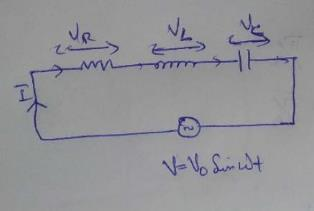V=ZI ->Impedance
I=V/Z
VR=IR=VR/Z=>R=(VR/V)Z
VL=IXL=VXL/Z=>XL=(VL/V)Z
Similarly, XL=(VC/V)Z
Impedance, Z2=R2+(XL-XC)2
=>Z2=(VR2/V2)Z2 +[{(VL/V)Z}-{(VC/V)Z}]2
V=√[VR2+(VL-VC)2 ]
On substituting, VL =3VR   , VC=2VR
=>V=√2 VR

QUESTION: 21

The percentage increase in the impedance of an ac circuit, when its power factor changes form 0.866 to 0.5 is (Resistance constant)

Solution:

Power factor (cosϕ)=R/Z1
For first case, power factor=0.866
Or, R/ Z1=0.866
Or,Z1=1.154R
For second case, power factor=>0.5
Cosϕ2=>R/Z2=>0.5, Z2=2R.
% increase=>[(Z2-Z1)/Z1] x100
So, [{(2R)-(1.154R)}/(1.154R)]x100=73.2%
So the correct answer is option A.

QUESTION: 22

In a series resonant L_C_R circuit, if L is increased by 25% and C is decreased by 20%, then the resonant frequency will

Solution:

v0=1/2π√(LC)
⇒In this question,
L’=L+25% of L
=L+(L/4)=5L/4
And C’=C-20% of C
=C-(C/5)=4C/5
Hence, v0=1/2π√(L’C’)
=1/2π√[(5L/4) x (4C/5)]
=1/2π√(LC)=v0
The answer is option C, remains unchanged.

QUESTION: 23

Which of the following statements is correct for L_C_R series combination in the condition of resonance

Solution:

Xnet(reactance)=XL-XC=0, =>XL=XC
ω=1/√(LC)
Z=√(R2 + (Xnet)2)

QUESTION: 24

An ac circuit resonates at a frequency of 10 kHz. If its frequency is increased to 11 kHz, then

Solution:

In an LCR ac circuit,
Impedance, Z=R+j(XL​−XC​) . . . . . . ..  .(1)
At the resonance, inductive reactance is equal to the capacitive reactance.
XL​=XC​
From equation (1),
Z=R
The impedance is independent of the frequency term.
On increasing the frequency, the impedance will remain unchanged.
The correct option is B.

QUESTION: 25

In an ac circuit emf and current are E = 5 cos wt volt and I = 2 sin wt ampere respectively. The average power dissipated in this circuit will be

Solution:

The voltage can be written as,
V=5(sinωt+(π/2​))
The angle between the voltage and the current is 90O.
The power dissipated in the circuit is given as,
P=Vrms​Irms​cosϕ
=Vrms​Irms​cos90O
=0
Thus, the power dissipated in the circuit is 0.

QUESTION: 26

A choke coil of negligible resistance carries 5 mA current when it is operated at 220 V. The loss of power in the choke coil is –

Solution:

Choke Coil=inductor
Φ=90o
P=VIcos Φ
=VIcos90o
=0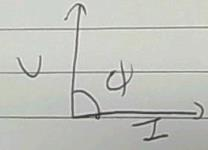QUESTION: 27

In an A.C. circuit, a resistance of 3W, an inductance coil of 4W and a condenser of 8W are connected in series with an A.C. source of 50 volt (R.M.S.). The average power loss in the circuit will be

Solution:
QUESTION: 28

Two bulbs of 500 watt and 300 watt work on 200 volt r.m.s. the ratio of their resistances will be –

Solution:

First bulb= v=220v
P=500w
P1= (v2/R1)
R1= V2/P1= 220x200/500 ohms
For second bulb:
v=220 v
P=300W
R2= v2/p=220x200/300
By taking ratios:
R1:R2= 220x200x 300: 500 x220x200 = 3:5

QUESTION: 29

Three bulbs of 40, 60 and 100 watt are connected in series with the source of 200 volt. Then which of the bulb will be glowing the most –

Solution: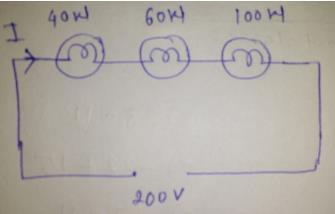Resistance= (voltage)2/Power
Resistance of 40 W bulb= (200)2/40=1000 ohm
Resistance of 60 W bulb=(200)2/60=666.67 ohm
Resistance of 100 w bulb=(200)2/100=400 ohm
Total resistance= 1000+666.67+400=2066.67 ohm (addition because series circuit)
Now, we calculate current flowing in the bulbs (as we know this is series circuit so same current will flow in the circuit)
Current (I)= Voltage/total resistance=200/2066.67=0.0967 A
Now we find the power consumed by each bulb,
power consumed by 40 W bulb=I2R=(0.0967)2x1000=9.35 W
Power consumed by 60 W bulb=I2xR=(0.0967)2x666.67=6.23 W
Power consumed by 100 W bulb=I2R=(0.0967)2x400=3.74 W
From the above results, we can say that power consumed by a 40 W bulb is more as compared to other bulbs so 40 W glows brighter than other bulbs.

QUESTION: 30

Alternating current can not be measured by direct current meters, because –

Solution:

The alternating current changes its direction and magnitude, it becomes zero twice in a complete cycle. In one half cycle current flows in one direction and changes its direction for other half cycle. So, it is not possible by direct current meters to read such a high fluctuation in a second due to inertia and hence, no deflection accounted. Hence, alternating current can not be measured by direct current meters, because the average value of current for the complete cycle is zero.